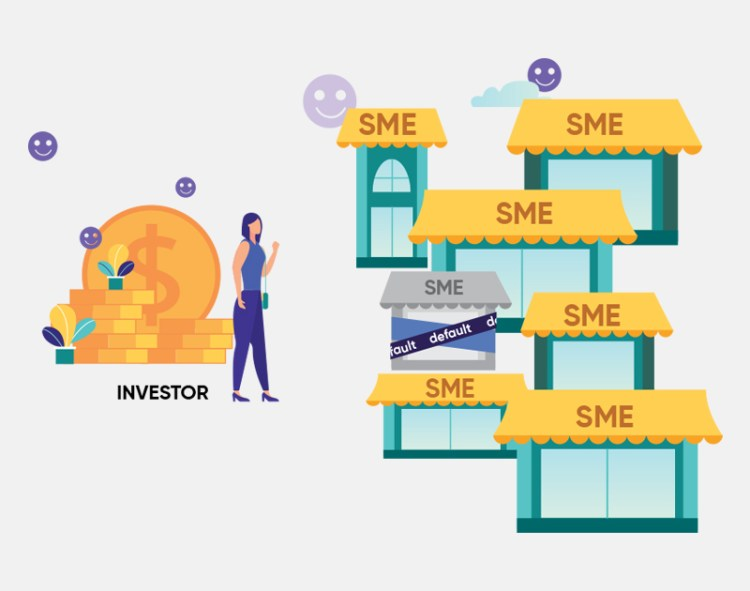One of the largest risks in investing in a Peer-to-Peer lending platform like Funding Societies is the risk of a loan defaulting. For business term loans, a default is defined as a loan that has been unpaid for over 90 days. For invoice financing, a default is defined as a loan that has been unpaid for over 60 days.

To manage the default risk, investors should consider diversifying their portfolios.

## What is diversification?

In investing, diversification refers to investing in multiple assets to reduce the risk of the portfolio. In the context of Peer-to-Peer investments, diversification refers to investing in multiple loan products.

### Invest in each and every loan

By investing the minimal amount into each and every loan opportunity offered, your risk is reduced. In the case where a loan was to default, the principal amount lost would not impact the entire portfolio heavily.

## How Does Diversification Work?

Illustration:
If an investor were to invest \$5000 into a single loan at 12% per annum with a tenor of 1 year, he would expect to earn \$600 in interest.
Total Interest Earned = 0.12 × \$5000 = \$600

However, in the unfortunate case that this single loan was to default, his total loss would be \$5000, with a rate of return of -100%.
Rate of Return = -\$5000 / \$5000 = – 100%If the same investor were to invest \$100 into 50 loans each at a 12% per annum with a tenor of 1 year, he would invest a total of \$5000 and also expect to earn \$600 in interest.
Total Interest Expected = 0.12 × \$5000 = \$600Assuming a 2% default rate, (which means that out of the 50 loans, 1 loan defaults) the investor would earn interest on the remaining 49 loans.
Total Interest Earned = 49 × \$100 × 0.12 = \$588

The investor loses \$100 from the default. The investors return on investment would therefore be:
Return on Investment = \$588 – \$100 = \$488

The rate of return, in this case, would then be:
Rate of Return = \$488 / \$5000= 9.76%

## Higher amount into lower yield and lower amount into higher yield

Depending on the risk appetite of the investor, some investors may prefer to invest more into lower yield loans as they are assessed to be stronger borrowers and smaller amounts into the higher yield loans.

A typical borrower could be charged between 8% – 11% p.a. in simple interest, while the ones assessed to have higher credit risk may pay interest at a higher bracket, between 12% – 16% p.a.

Illustration:
An investor invests \$1,000 into 3 loans at average 9% p.a. and \$100 into 20 loans at average 14% p.a.
The investor obtains \$270 from the lower yield loans and \$280 from the higher yield loans for a total portfolio gross return of \$550. Assuming a 3% default on principal, your portfolio would still yield \$400 in gross returns.